Appended Note 1-4 Estimation of labor share and equilibrium level

1. Concept
It is thought that labor share goes through (1) long-term equilibrium, (2) shift from the equilibrium, and (3) a period which reflects the short-term economic cyclic process. In other words, the change in labor share in the economic cycle is considered as the correction process of the degree of deviation from the equilibrium of the real wage and labor productivity while deeming the relationship between these two factors as being at equilibrium.
Labor share = WL/PY = W/P(Real wage)*L/Y(reciprocal number of productivity)...(a)

2. Estimation Method
The fact that the real wage and labor productivity may be plotted using the error correction model (ECM) is equivalent to the two factors holding a co-integrated property. In order for these two factors to hold a co-integrated property, it is necessary for the two factors to have the following qualities: (1) the standard series to have a probabilistic trend and (2) the difference series to be stationary. Therefore, the two factors were first checked for their co-integrated property.
Upon conducting an ordinary unit root test, the results were inconsistent with the logic, giving incoherent results when divided into periods. There is a possibility that consistent results were not attained, given that structural changes is not accounted for in an ordinary unit root test. As such, unit root test was conducted following the method proposed by Perron in 1989 which accounts for the possibility of structural changes. As a result, the series of the real wage and labor productivity satisfied both qualities (1) and (2), verifying that the two factors both fulfilled the conditions that serve as the premise of holding a co-integrated property.
Next, the relationship of the two factors were defined as described in formula (b) below in order to test for a co-integrated property between the real wage and labor productivity. Then conducted was an analysis for co-integrated property proposed by Gregory and Hansen in 1996 which accounts for the possibility of structural changes. As a result, the null hypothesis was rejected and a co-integrated property was recognized between the two factors. Furthermore, the point of structural changes was in 1975 Term IV and level shift (change in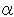) and trend shift (change in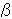) was detected at the time. When estimating the vector for co-integration while determining 1975 Term IV as the point of structural changes, each parameter and dummy variable representing the structural changes was of a significant value, providing that the structural changes took place in 1975 Term IV.
ln (W/P) =+*ln (Y/L)......(b)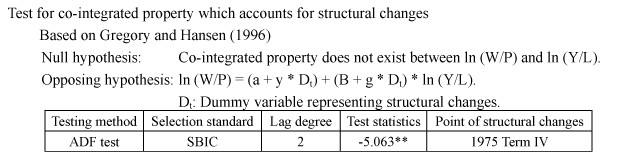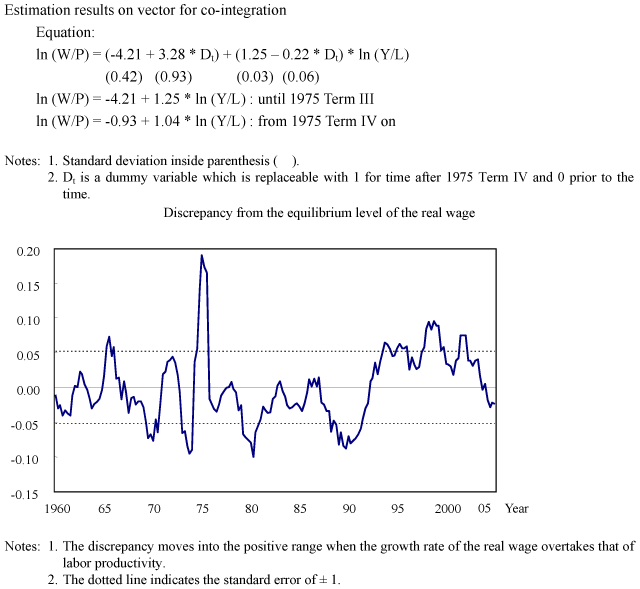The equilibrium labor share for Figure 1-1-17 is attained by substituting the estimated value from the above equilibrium formula with (a).

3. Definition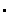Estimation period:1960 Term I - 2005 Term IAnnual Report on National Accounts, Cabinet Office; Statistics of Corporations by Industry, Quarterly, Ministry of Finance (all sizes in all industries (Capital of over 10 million yen))Labor share = Labor cost/(Labor cost + Operating profit + Depreciation charges)Real wage = Labor cost/Number of persons employed/GDP deflatorLabor productivity = (Labor cost + Operating profit + Depreciation charges)/GDP deflator/Number of persons employed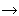Labor share, real wage and labor productivity are seasonally adjusted with X-11.